# DAV Class 4 Maths Chapter 9 Worksheet 7 Solutions

The DAV Class 4 Maths Solutions and DAV Class 4 Maths Chapter 9 Worksheet 7 Solutions of Fractions offer comprehensive answers to textbook questions.

## DAV Class 4 Maths Ch 9 WS 7 Solutions

Question 1.
Change into mixed number.
(a) $$\frac{4}{3}$$
$$\frac{4}{3}$$
= 4 ÷ 3
= 1$$\frac{1}{3}$$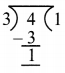(b) $$\frac{41}{10}$$
$$\frac{41}{10}$$
= 41 ÷ 10
= 4$$\frac{1}{10}$$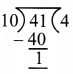(c) $$\frac{11}{4}$$
$$\frac{11}{4}$$
= 11 ÷ 4
= 2$$\frac{3}{4}$$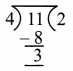(d) $$\frac{29}{6}$$
$$\frac{29}{6}$$
= 29 ÷ 6
= 4$$\frac{5}{6}$$(e) $$\frac{7}{5}$$
$$\frac{7}{5}$$
= 7 ÷ 5
= 1$$\frac{2}{5}$$(f) $$\frac{50}{9}$$
$$\frac{50}{9}$$
= 50 ÷ 9
= 5$$\frac{5}{9}$$(g) $$\frac{68}{11}$$
$$\frac{68}{11}$$
= 68 ÷ 11
= 6$$\frac{2}{11}$$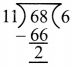(h) $$\frac{74}{15}$$
$$\frac{74}{15}$$
= 74 ÷ 15
= 4$$\frac{14}{15}$$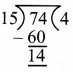(i) $$\frac{54}{7}$$
$$\frac{54}{7}$$
= 54 ÷ 7
= 7 $$\frac{5}{7}$$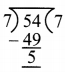Question 2.
Change into improper fractions.
(a) 2$$\frac{1}{3}$$
= (2 × 3) + 1
= 6 + 1 = 7
$$\frac{7}{3}$$

(b) 6$$\frac{1}{4}$$
= (4 × 6) + 1
= 24 + 1 = 25
= $$\frac{25}{4}$$

(c) 1$$\frac{4}{9}$$
= (1 × 9) + 4
= 9 + 4 = 13
= $$\frac{13}{9}$$

(d) 33$$\frac{1}{3}$$
= (33 × 3) + 1
= 99 + 1 = 100
= $$\frac{100}{3}$$

(e) 10$$\frac{1}{10}$$
= (10 × 10) + 1
= 100 + 1 = 101
= $$\frac{101}{10}$$

(f) 7$$\frac{5}{6}$$
= (7 × 6) + 5
= 42 + 5 = 47
= $$\frac{47}{6}$$

(g) 12$$\frac{3}{7}$$
= (12 × 7) + 3
= 84 + 3 = 87
= $$\frac{87}{7}$$

(h) 9$$\frac{1}{9}$$
= (9 × 9) + 1
= 81 + 1 = 82
= $$\frac{82}{9}$$

(i) 4$$\frac{7}{8}$$
= (4 × 8)
= 32 + 7 = 39
= $$\frac{39}{8}$$

### DAV Class 4 Maths Chapter 9 Worksheet 7 Notes

Changing Fractions
1. Imprper fractrion into mixed number.
E.g. Change $$\frac{17}{2}$$ into mixed number.
$$\frac{17}{2}$$ can be written as 17 ÷ 2so $$\frac{17}{2}$$ = 8$$\frac{1}{2}$$
E.g. Change 2$$\frac{1}{2}$$ into fraction.
2$$\frac{1}{3}$$ = 2 × 3 = 6
2$$\frac{1}{3}$$ = 6 + 1 = $$\frac{7}{3}$$